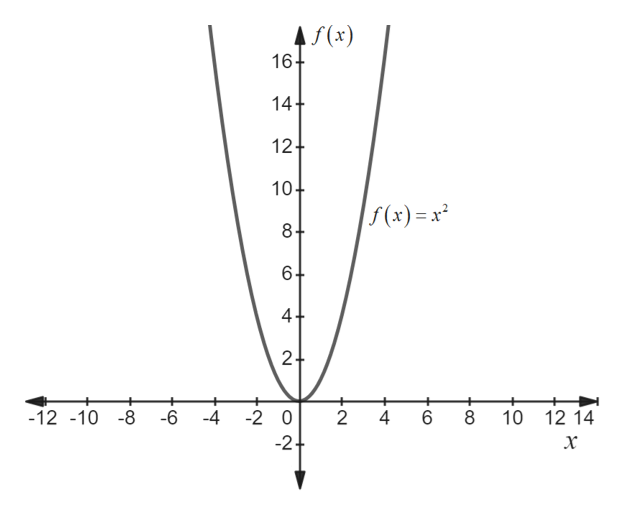Let y(x) = ax2 + bx + c. The function y(x) is not invertible, however, like the function f(x) = x2 we can make some restrictions on its domain to make it invertible. Hint: Think of the example (x2) given above.Find the largest possible domain where y(x) is invertibleFind its inverse function using this domainCheck that it is indeed the inverse function by verifying the identity y−1(y(x)) =y(y−1(x)) = xShow limx→3 x2 −3x+1 = 1 using the definition of limit, that is, using ε and δ.

Question

Let y(x) = ax2 + bx + c. The function y(x) is not invertible, however, like the function f(x) = x2 we can make some restrictions on its domain to make it invertible. Hint: Think of the example (x2) given above.

• Find the largest possible domain where y(x) is invertible
• Find its inverse function using this domain
• Check that it is indeed the inverse function by verifying the identity y−1(y(x)) =y(y−1(x)) = x
• Show limx→3 x2 −3x+1 = 1 using the definition of limit, that is, using ε and δ.
Step 1

Hey, since there are multiple sub-parts posted, we will answer first three sub-parts. If you want any sub-parts to be answered, then please submit that sub-parts only or specify the sub-parts number in your message.

Step 2

a) First draw the graph for the given function as shown in below figure.help_outlineImage TranscriptioncloseAf(x) 16+ 14+ 12+ 10 f(x) x 8+ 6+ 4+ 2 + -12 -10 -8 -6 -4 -2 0 2 4 10 12 14 -2 x CO CO N fullscreen
Step 3

From the above graph, x can have 2 value for each value of y.

So, horizontal test failed and the function is not invertible.

Make the function invertible with the help of restricting the domai...

Want to see the full answer?

See Solution

Want to see this answer and more?

Our solutions are written by experts, many with advanced degrees, and available 24/7

See Solution
Tagged in

Functions